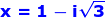Wednesday, December 3, 2014

Theory of Equations, 5

Category: Algebra

"Published in Newark, California, USA"

Find the remaining roots of the equation

if -2 is a root.

Solution:

If one of the root of the equation is given which is -2, then we need to get its conjugate because we want to eliminate the radical sign in the given equation. The conjugate of -2 is 2. Hence, the equation or a factor from the product of a root and its conjugate is

In order to get the other factor for the given equation, let's divide the given equation with the above equation, we have

Since there's no remainder in the division, then the other factor of the given equation is x² - 2x + 4.

By using the completing the square method, we can solve for the other roots of the given equation as follows

Therefore, the other roots of the given equation areand.